# NCERT Exemplar Class 10 Maths Solutions for Chapter 11 - Areas Related To Circles

## NCERT Exemplar Solutions Class 10 Maths Chapter 11 – Free PDF Download

NCERT Exemplar Class 10 Maths Chapter 11 Areas Related to Circles, provided here, in downloadable PDF format for students to prepare for CBSE exams. The material is prepared as per CBSE latest syllabus (2021-2022) and NCERT guidelines by our subject experts. It will help them to score better marks in the exam. These exemplars are prepared in PDF format so that students can download it and practice it offline as well. Students can use it to solve difficult problems taking this material as a reference tool and at the same time study effectively for the exams. Click here to get exemplars for all chapters.

Students can use NCERT Exemplars consisting of solved questions, to get a clear idea of the important formulas and how to apply them in finding the right solutions. These exemplars problems and solutions are designed by Maths experts with respect to CBSE Syllabus, which covers the following topics of Areas Related to Circles given below;

• Finding the Perimeter and Area of a Circle
• Finding the area of the sector and segment of a circle
• Finding the areas of Combinations of Plane Figures

## Download PDF of NCERT Exemplar Class 10 Maths Chapter 11 Areas Related to Circles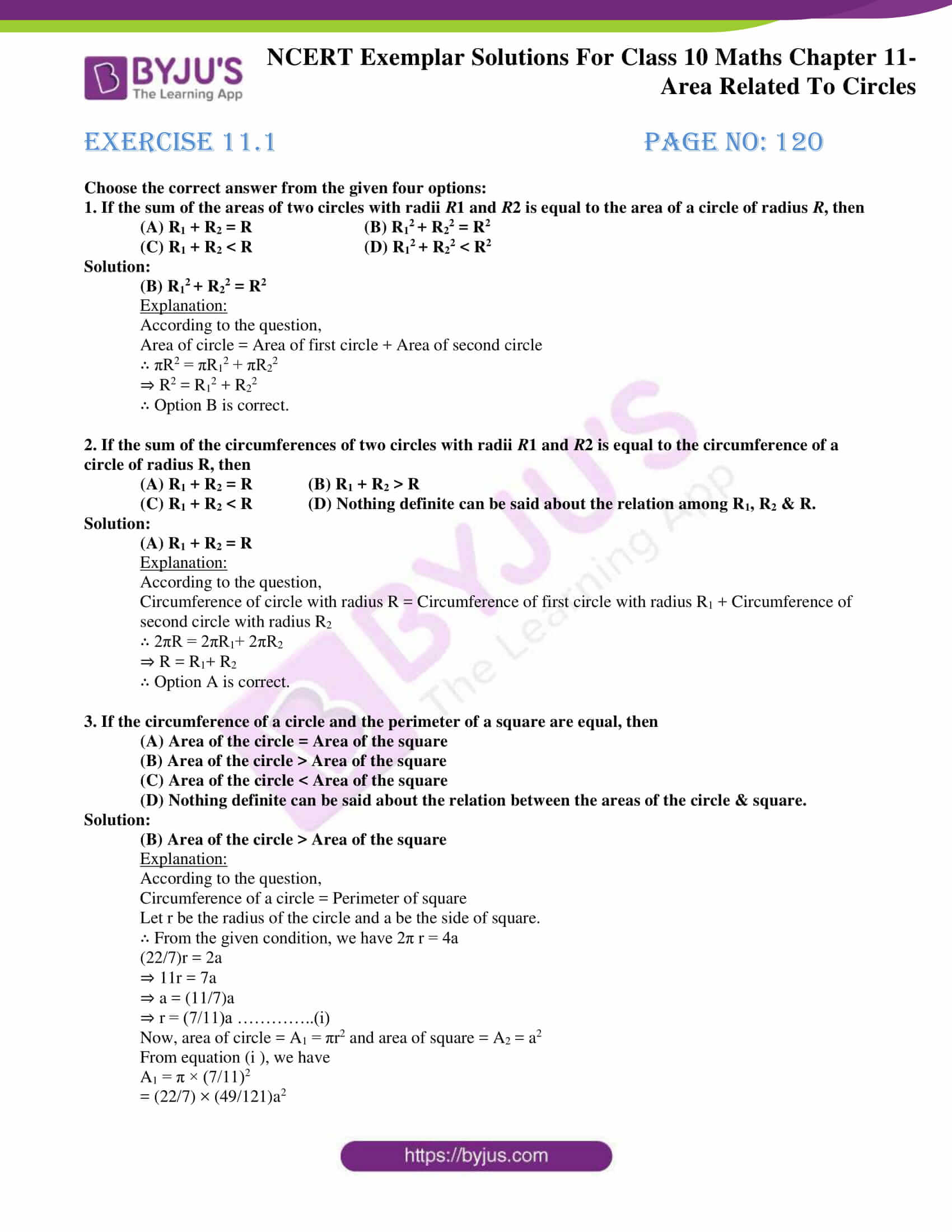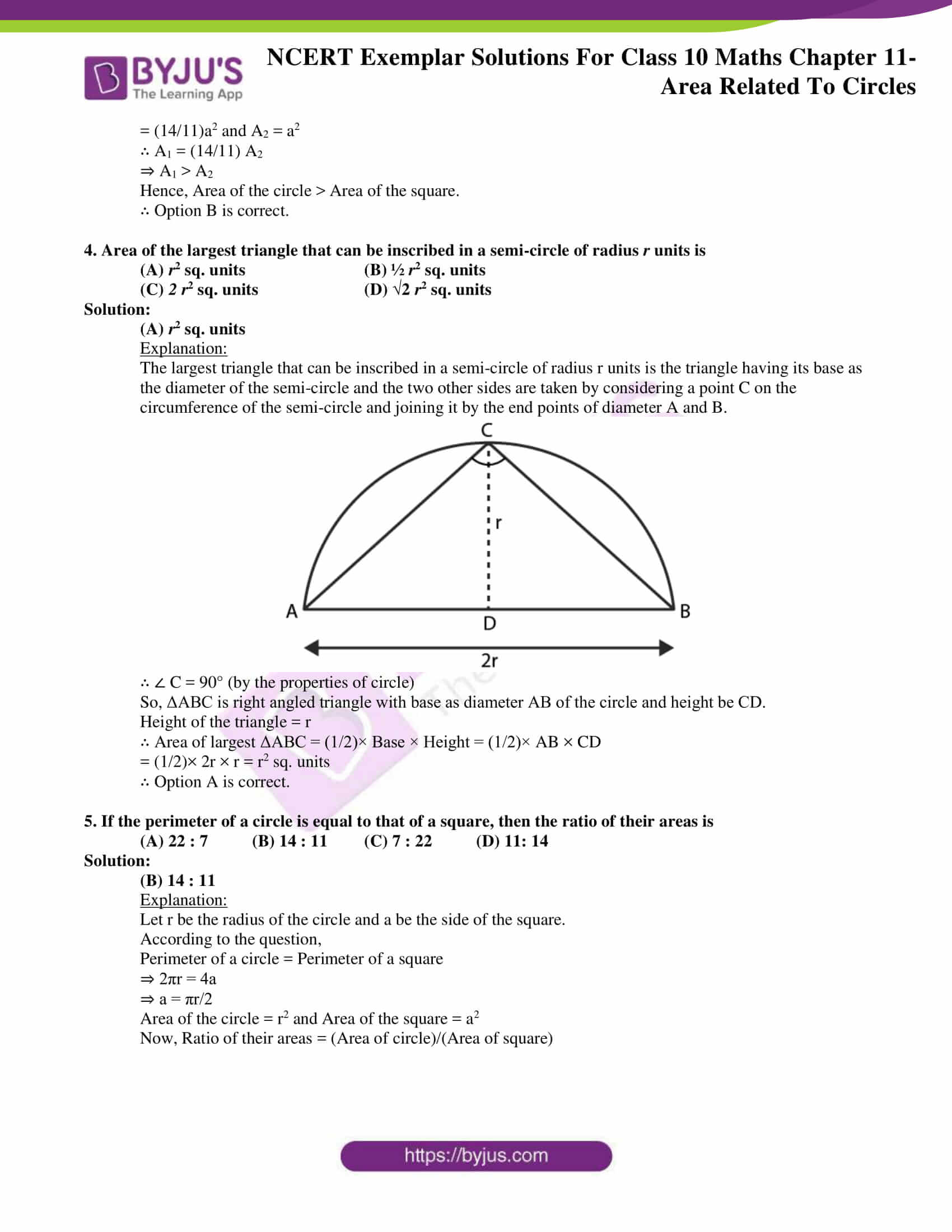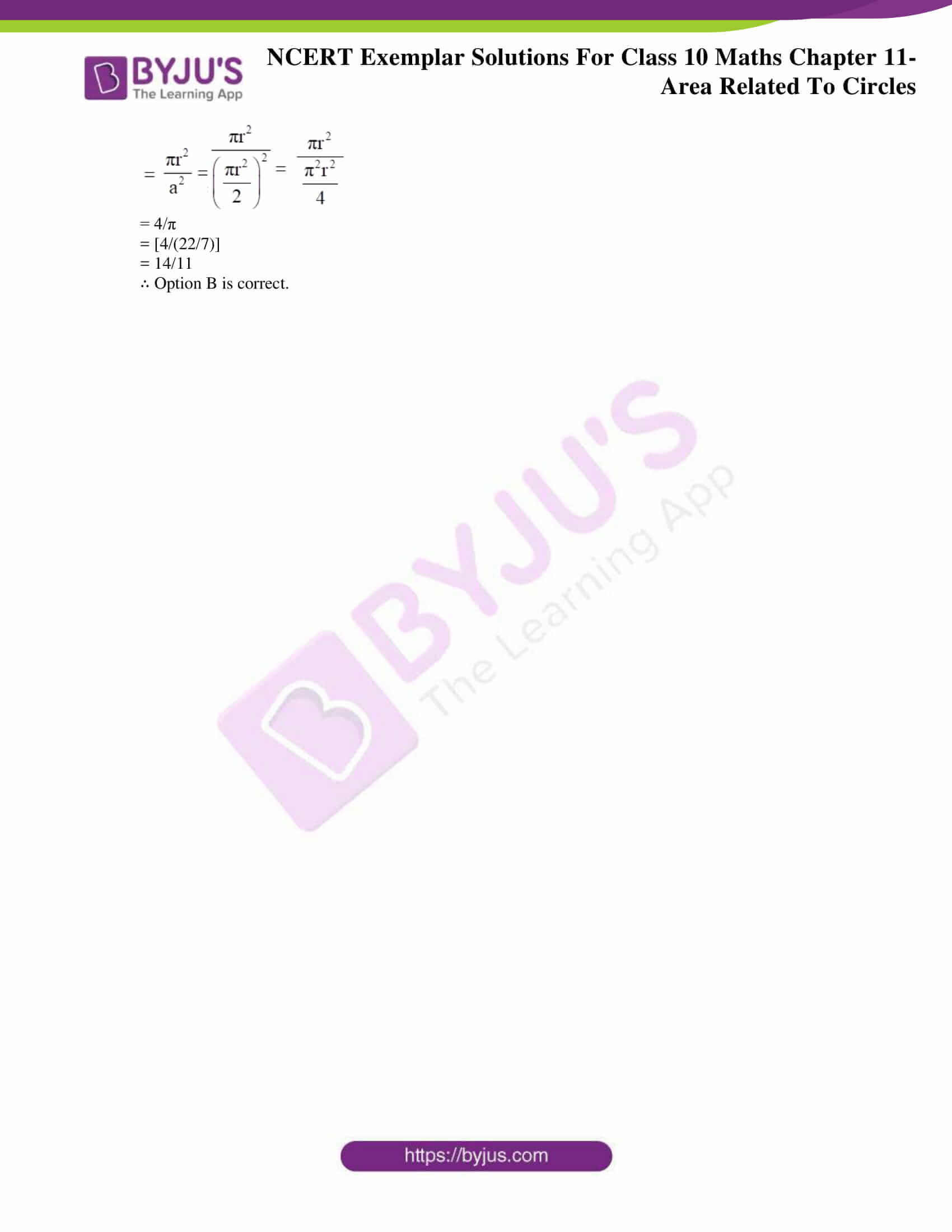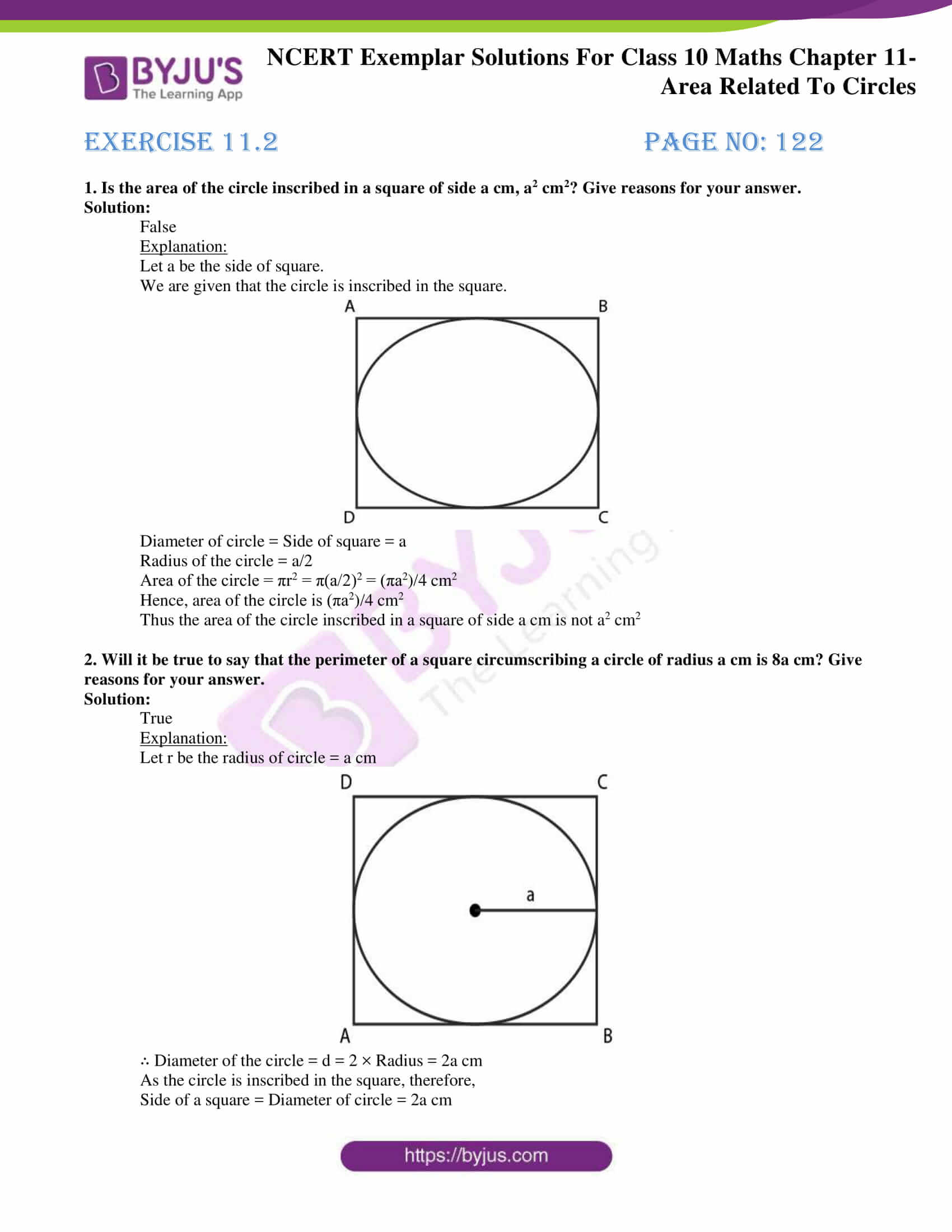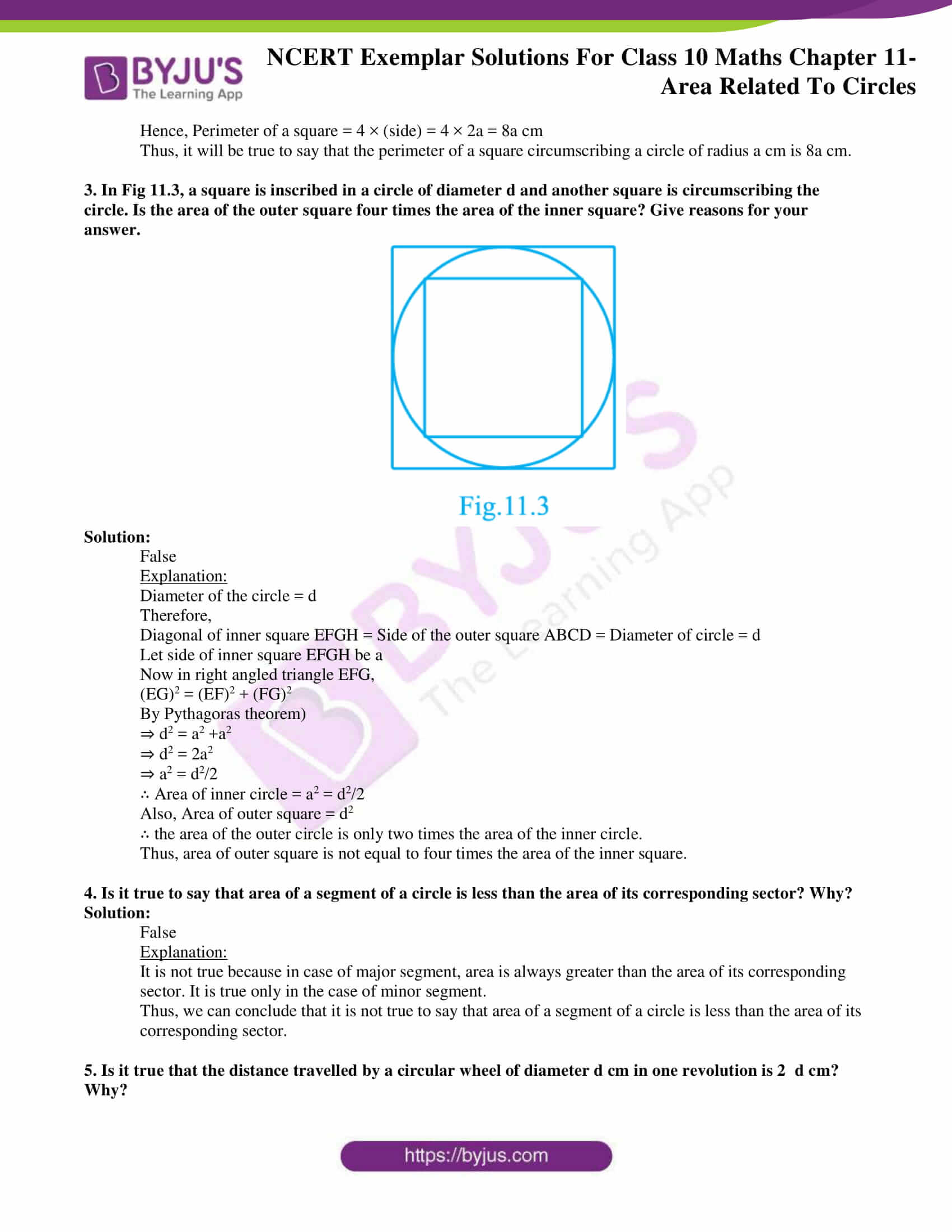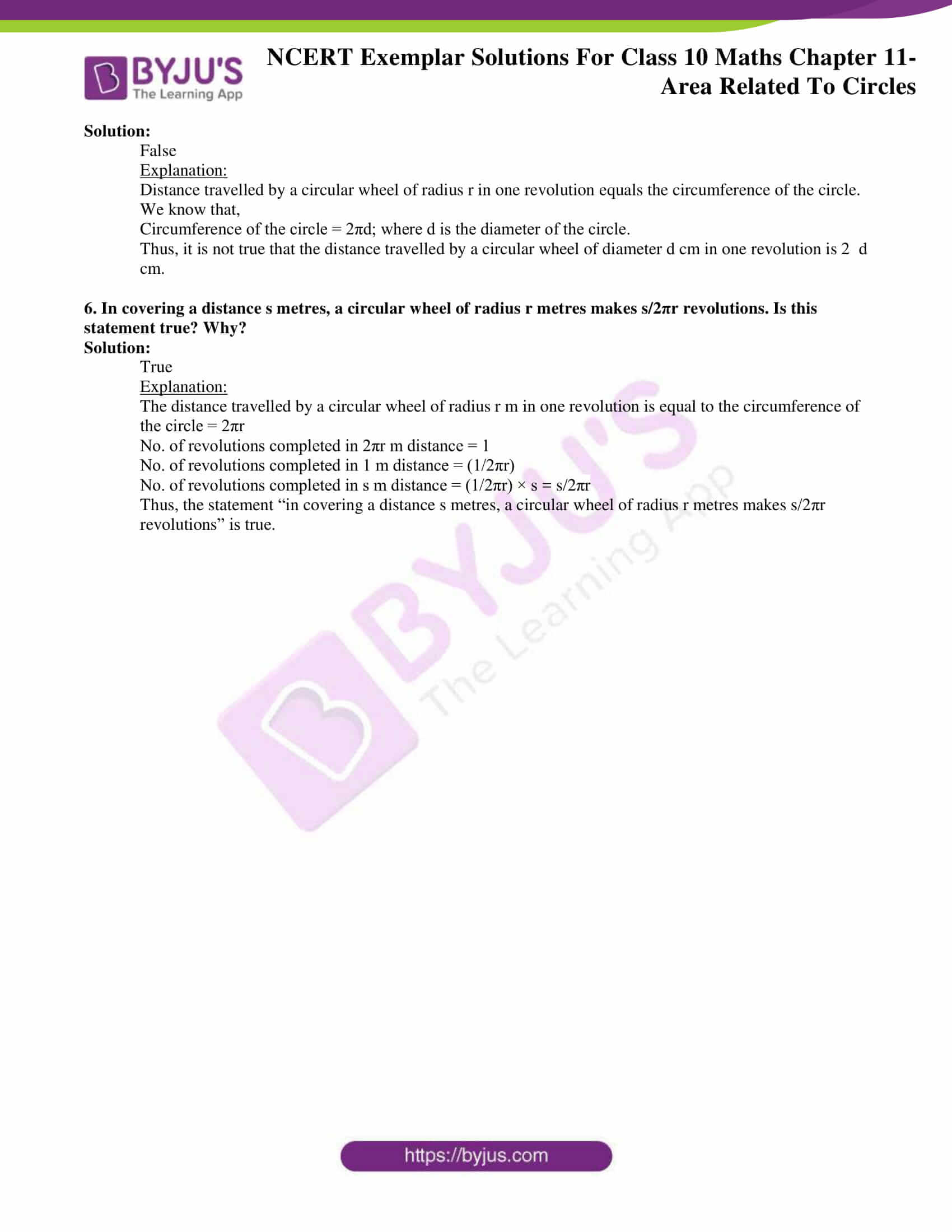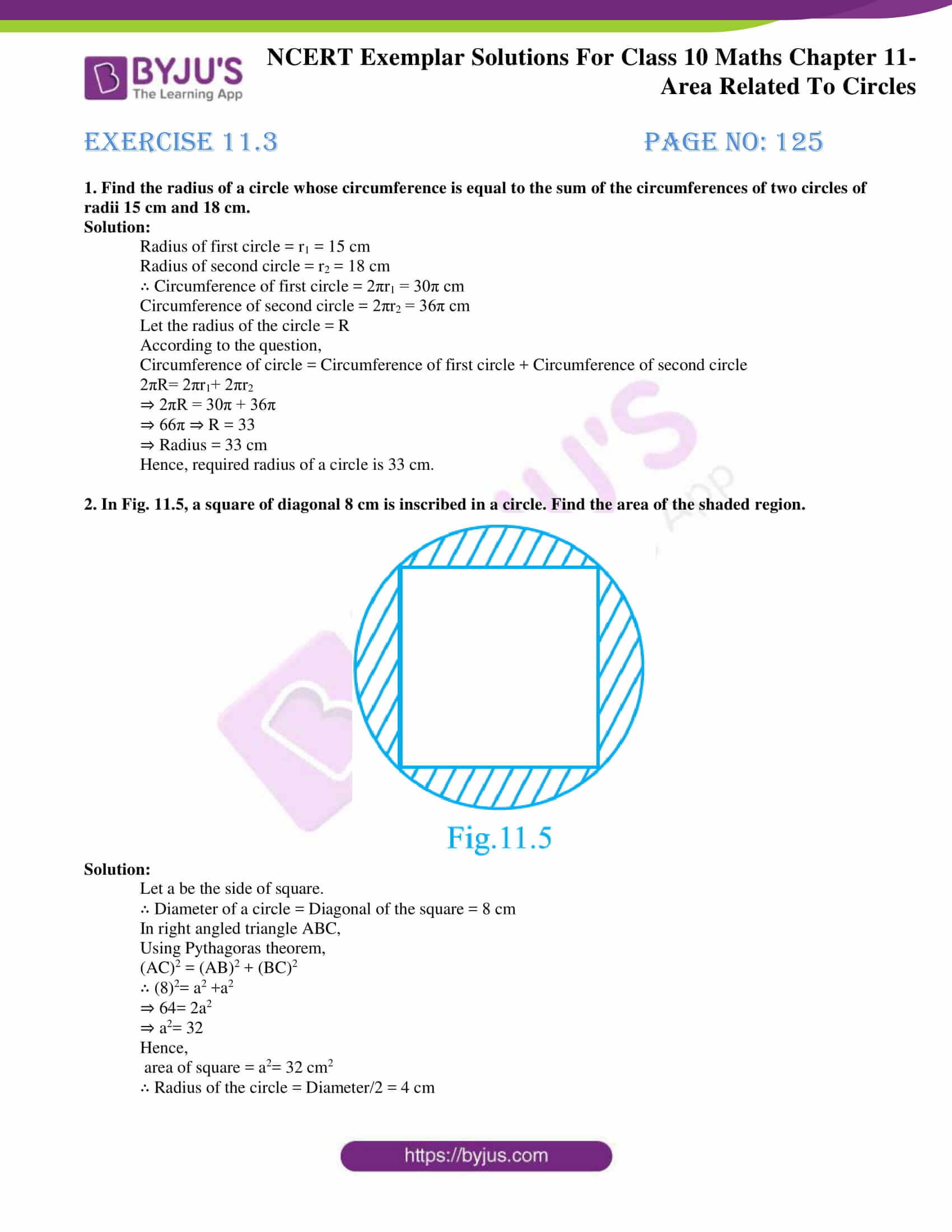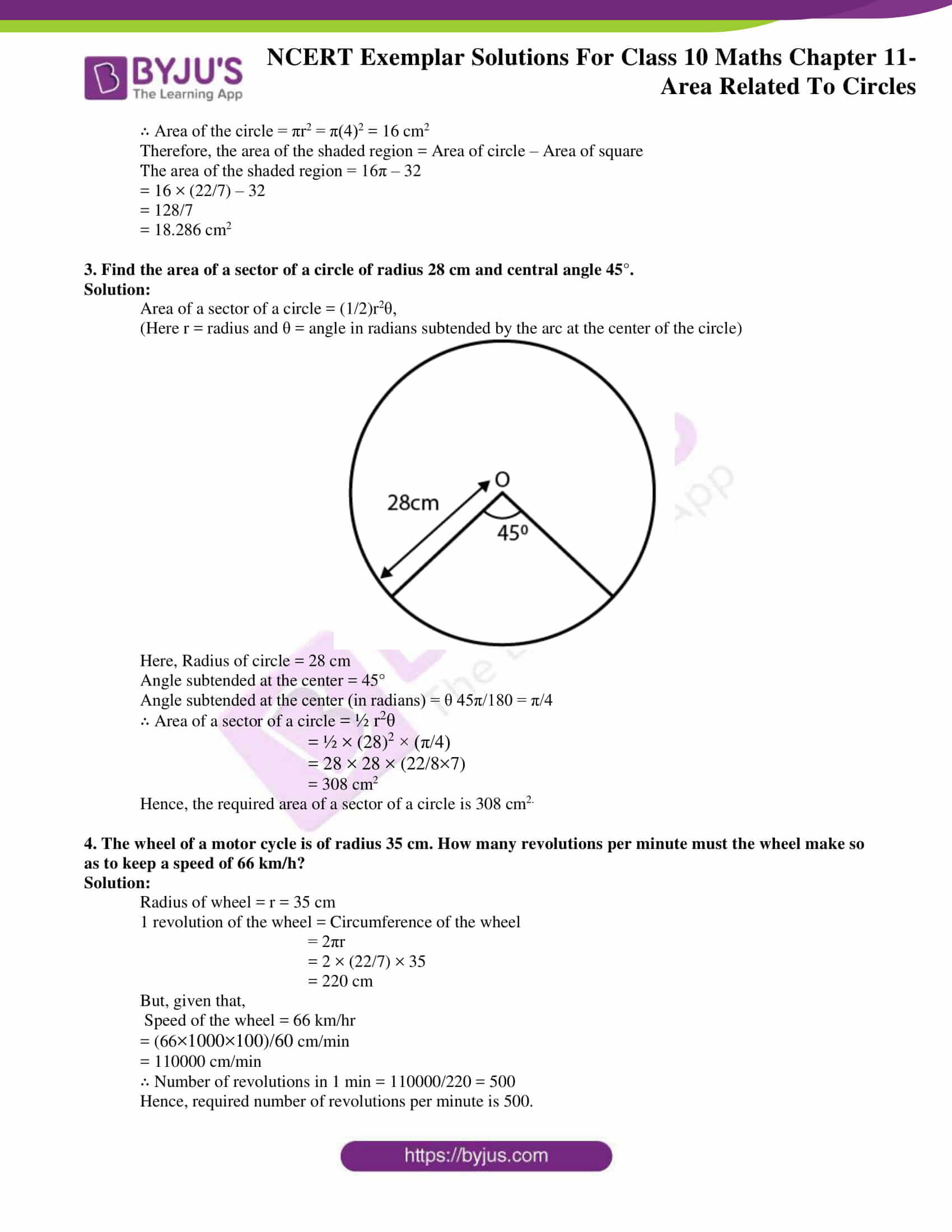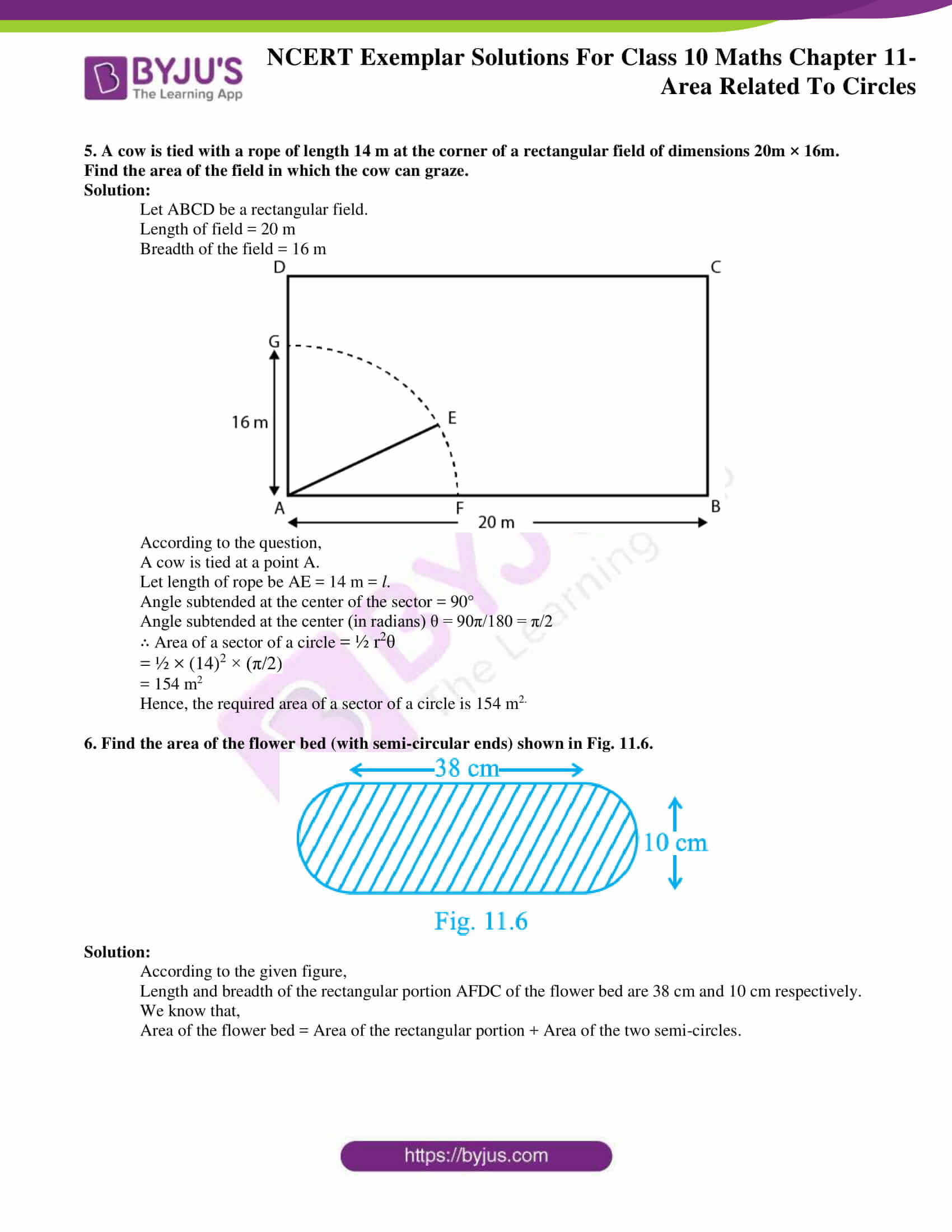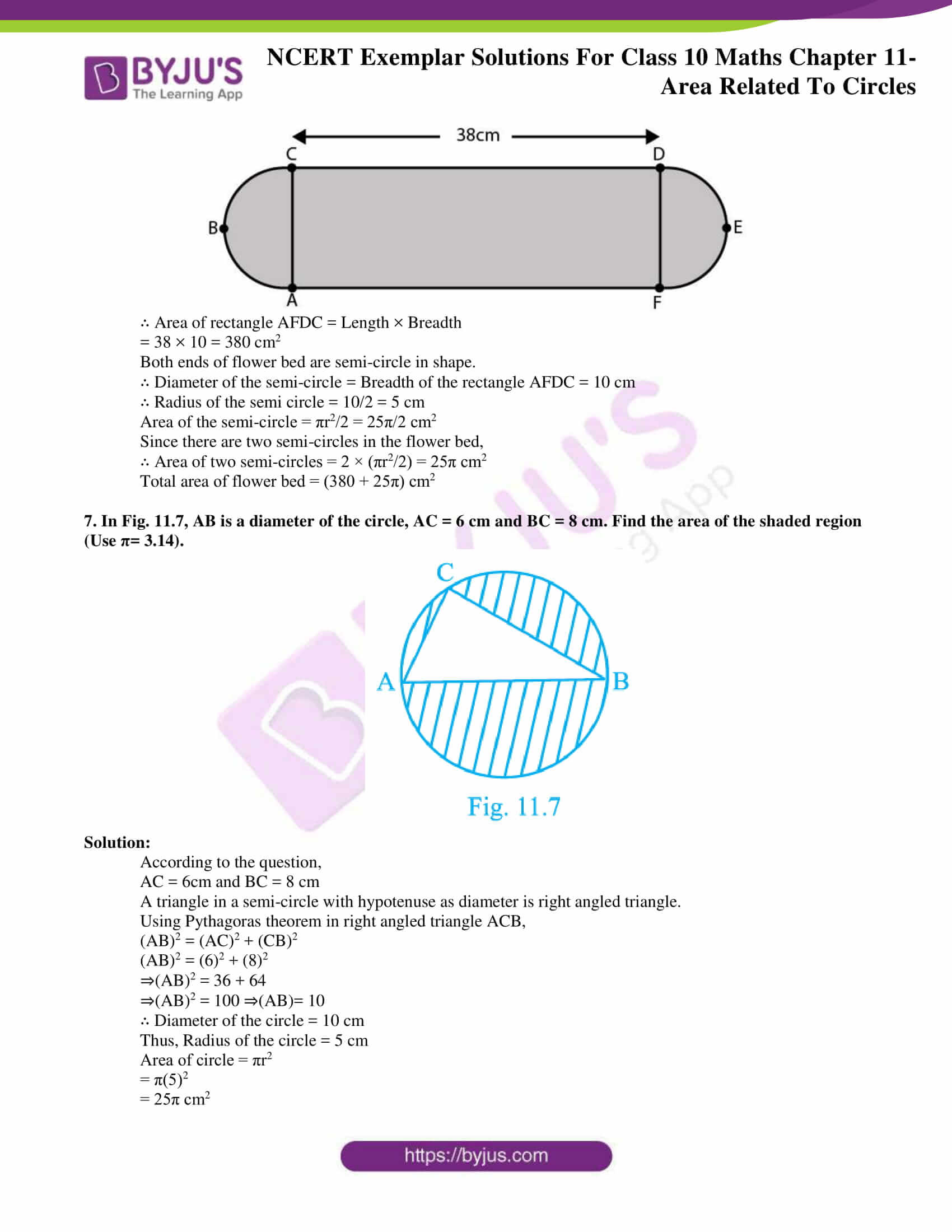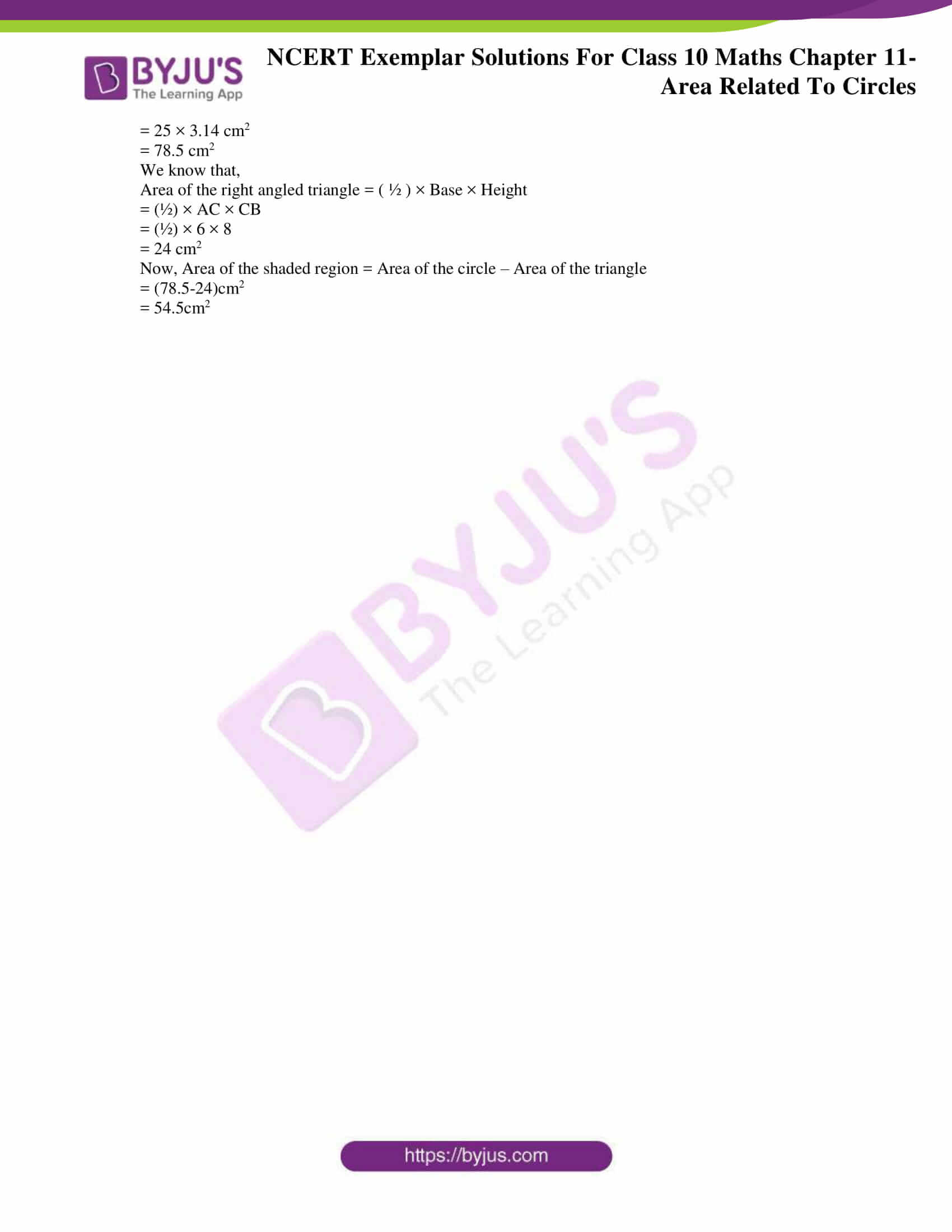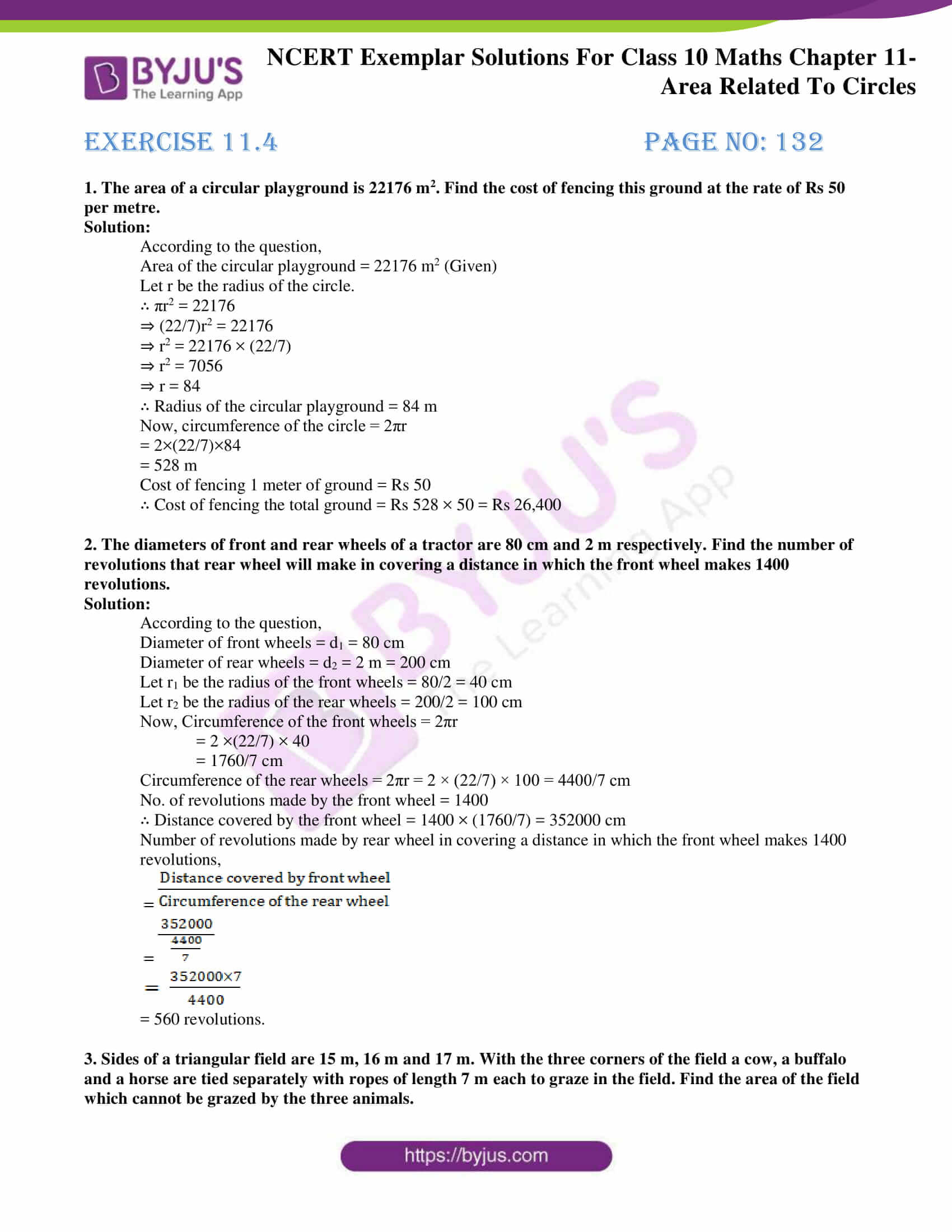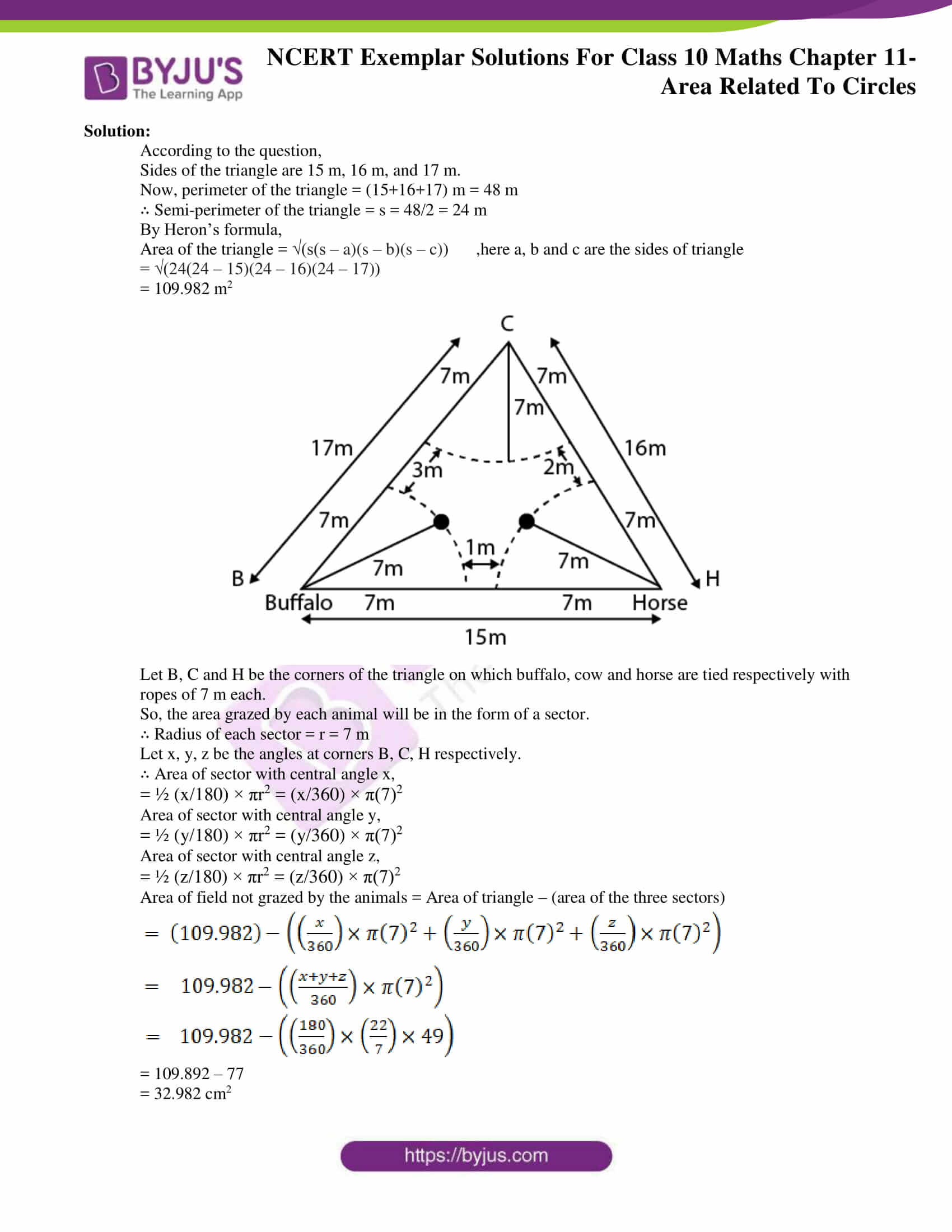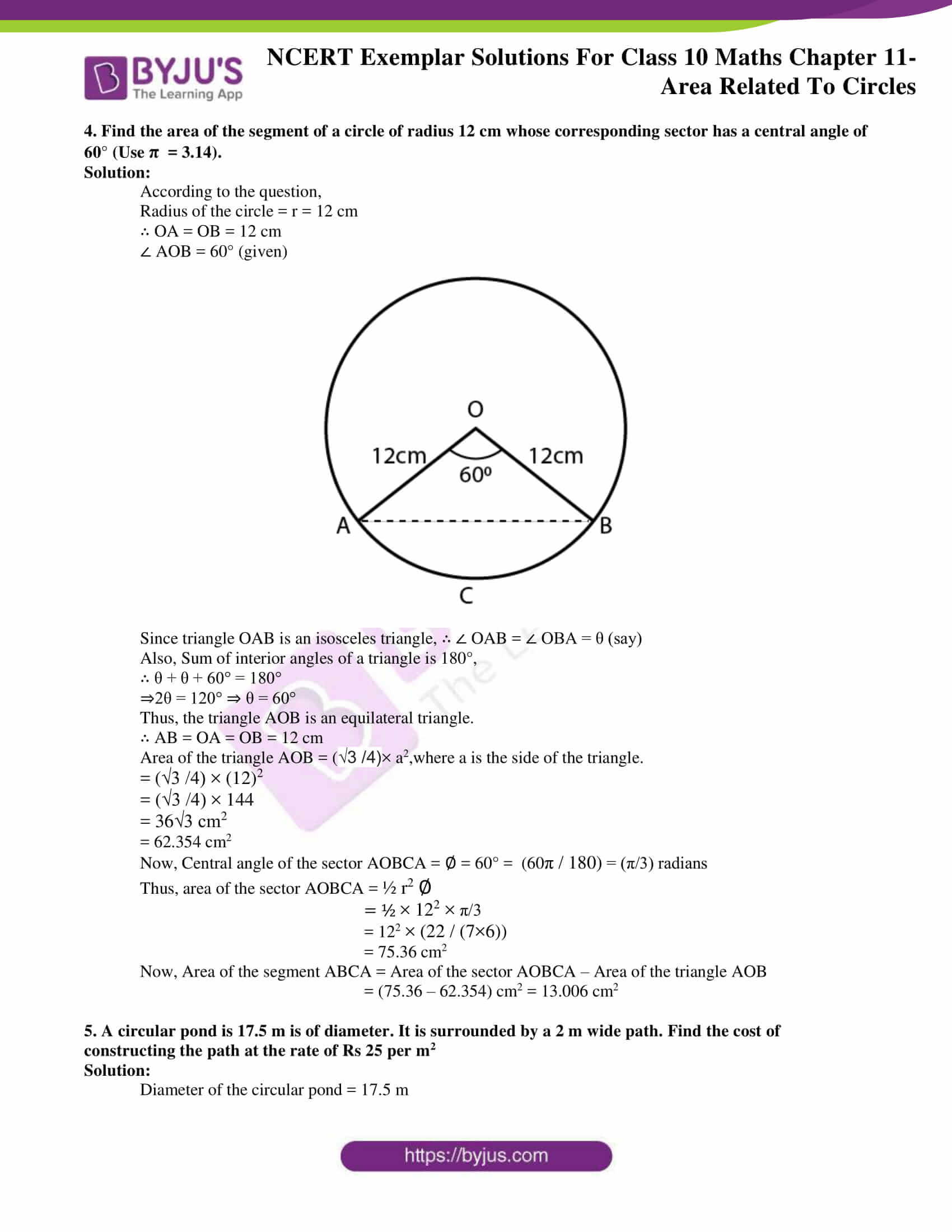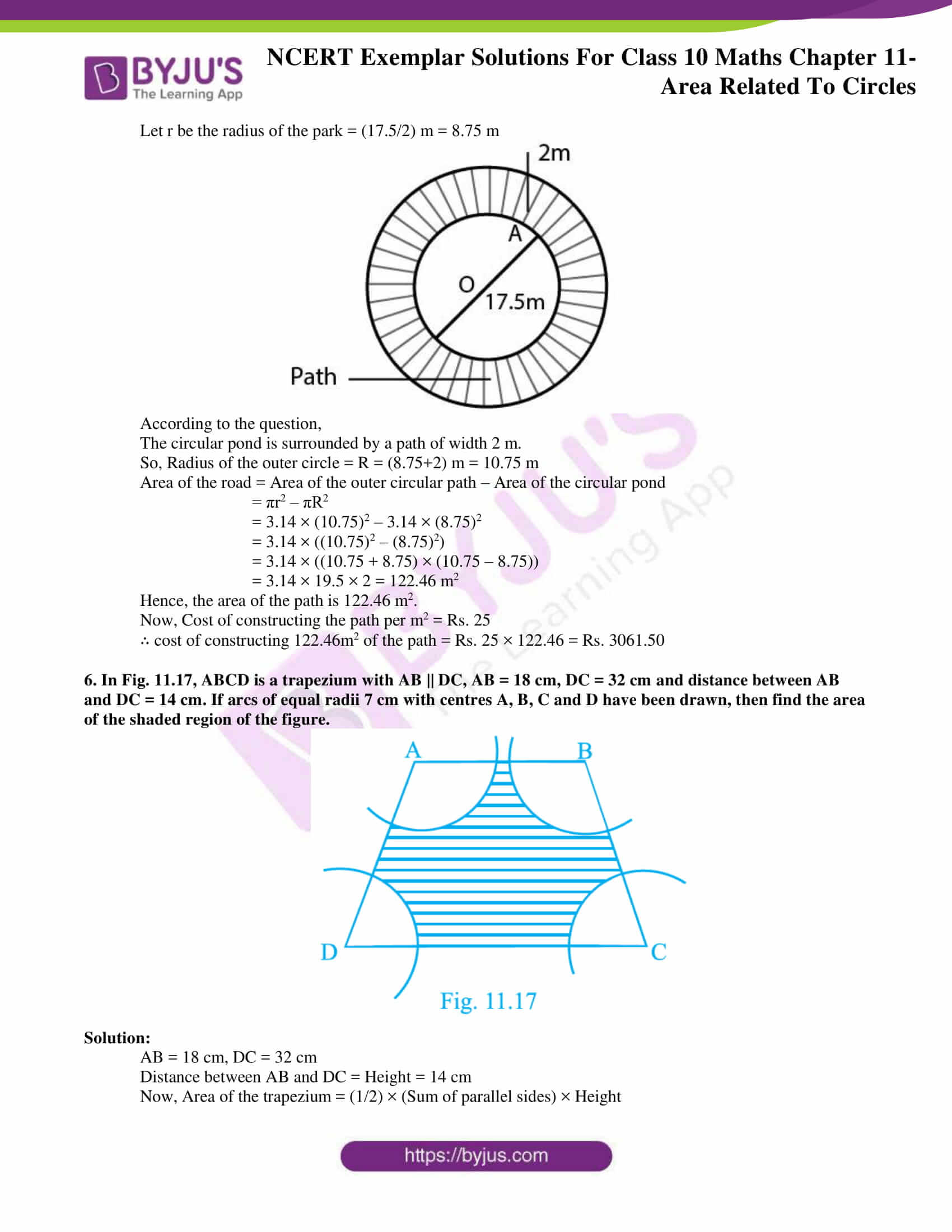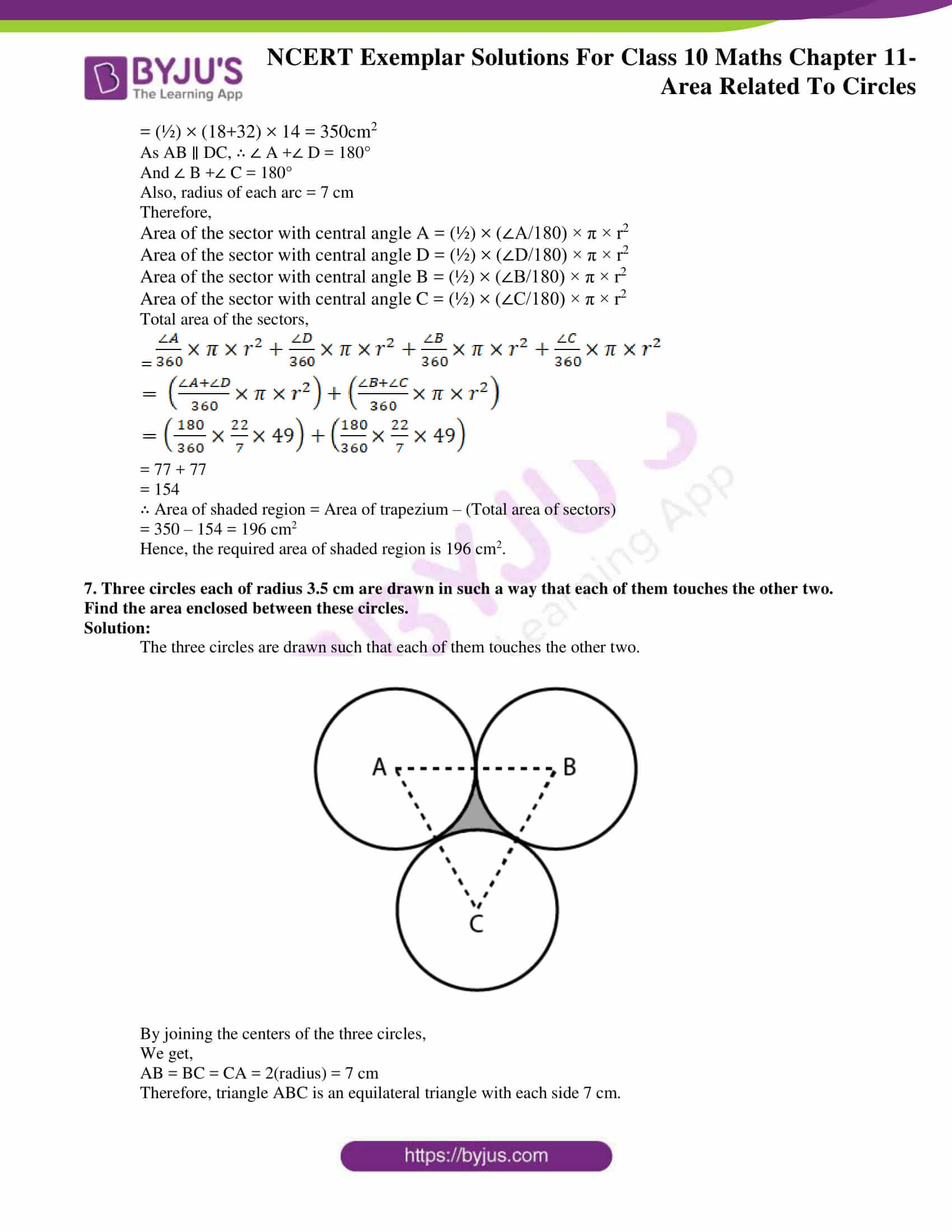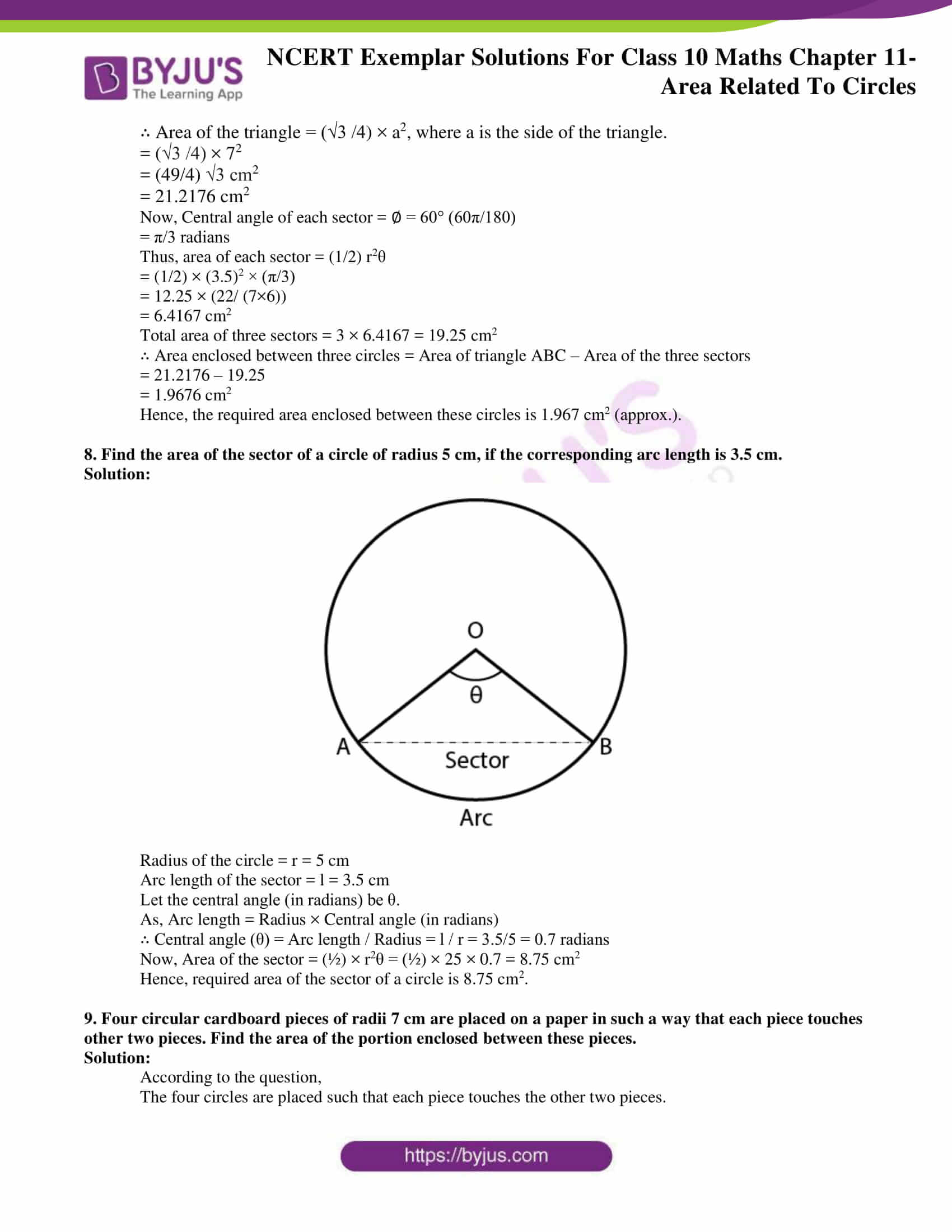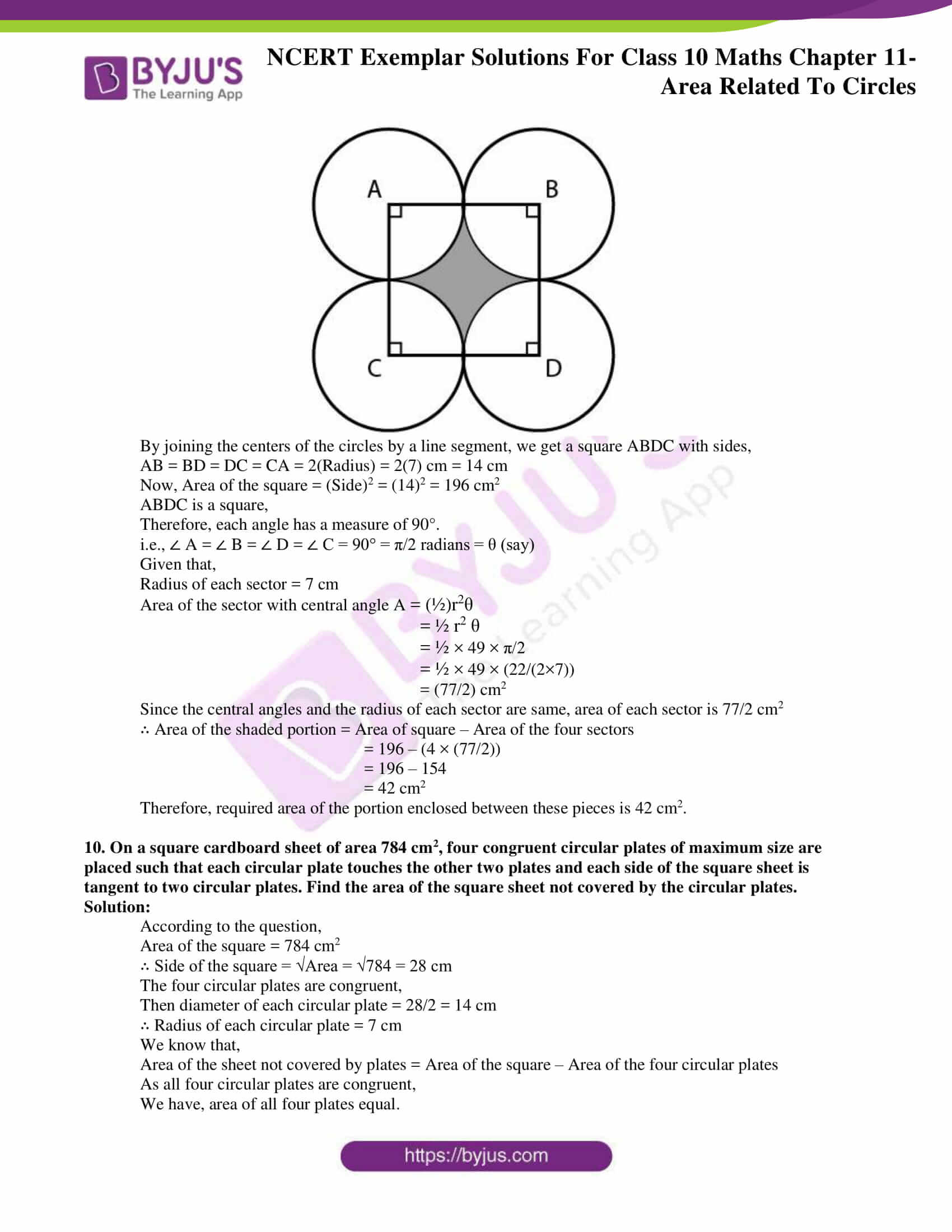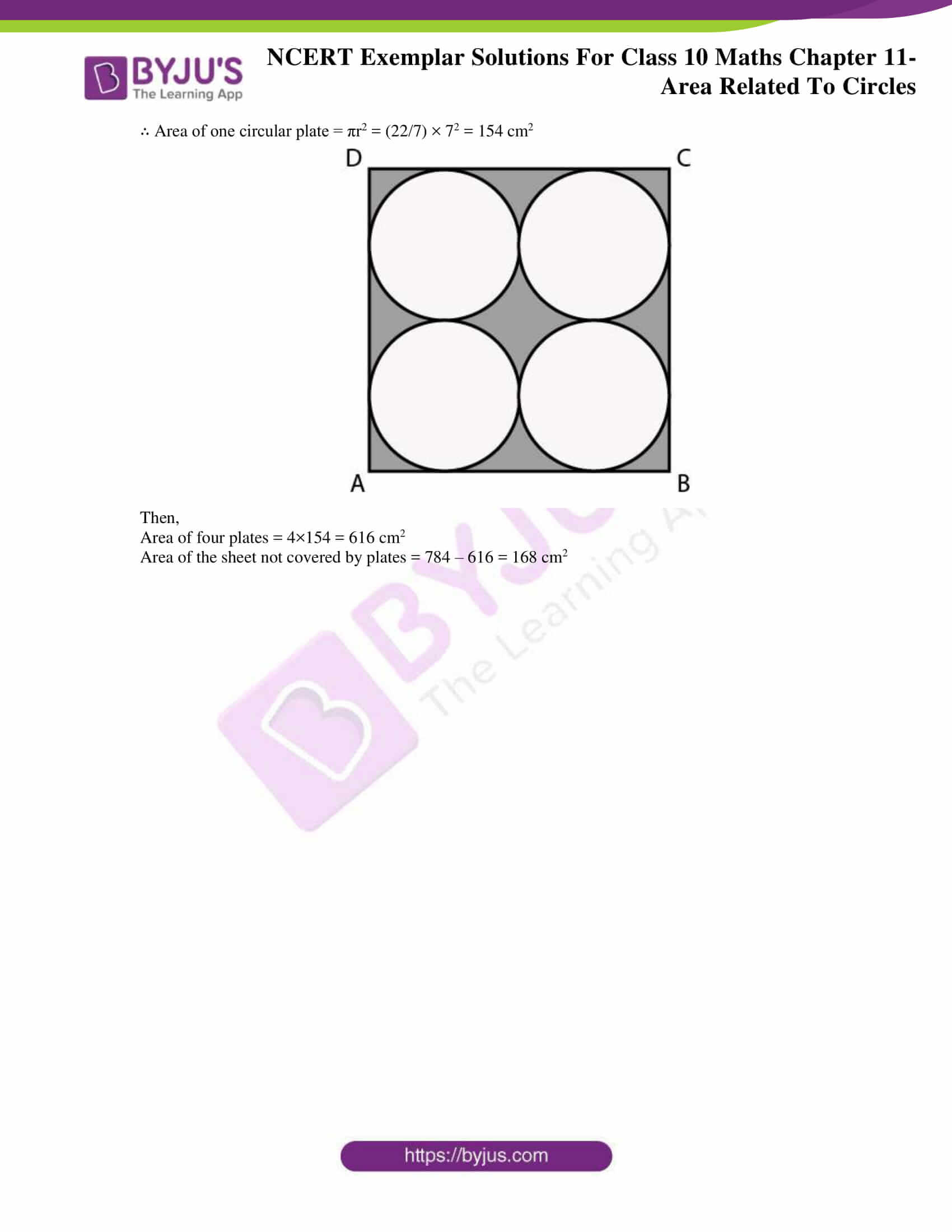## Exercise 11.1 Page No: 120

Choose the correct answer from the given four options:

1. If the sum of the areas of two circles with radii R1 and R2 is equal to the area of a circle of radius R, then

(A) R1 + R2 = R (B) R12 + R22 = R2

(C) R1 + R2 < R (D) R12 + R22 < R2

Solution:

(B) R12 + R22 = R2

Explanation:

According to the question,

Area of circle = Area of first circle + Area of second circle

∴ πR2 = πR12 + πR22

⇒ R2 = R12 + R22

∴ Option B is correct.

2. If the sum of the circumferences of two circles with radii R1 and R2 is equal to the circumference of a circle of radius R, then

(A) R1 + R2 = R (B) R1 + R2 > R

(C) R1 + R2 < R (D) Nothing definite can be said about the relation among R1, R2 & R.

Solution:

1. R1 + R2 = R

Explanation:

According to the question,

Circumference of circle with radius R = Circumference of first circle with radius R1 + Circumference of second circle with radius R2

∴ 2πR = 2πR1+ 2πR2

⇒ R = R1+ R2

∴ Option A is correct.

3. If the circumference of a circle and the perimeter of a square are equal, then

(A) Area of the circle = Area of the square

(B) Area of the circle > Area of the square

(C) Area of the circle < Area of the square

(D) Nothing definite can be said about the relation between the areas of the circle & square.

Solution:

(B) Area of the circle > Area of the square

Explanation:

According to the question,

Circumference of a circle = Perimeter of square

Let r be the radius of the circle and a be the side of square.

∴ From the given condition, we have 2π r = 4a

(22/7)r = 2a

⇒ 11r = 7a

⇒ a = (11/7)a

⇒ r = (7/11)a …………..(i)

Now, area of circle = A1 = πr2 and area of square = A2 = a2

From equation (i ), we have

A1 = π × (7/11)2

= (22/7) × (49/121)a2

= (14/11)a2 and A2 = a2

∴ A1 = (14/11) A2

⇒ A1 > A2

Hence, Area of the circle > Area of the square.

∴ Option B is correct.

4. Area of the largest triangle that can be inscribed in a semi-circle of radius r units is

(A) r2 sq. units (B) ½ r2 sq. units

(C) 2 r2 sq. units (D) 2 r2 sq. units

Solution:

(A) r2 sq. units

Explanation:

The largest triangle that can be inscribed in a semi-circle of radius r units is the triangle having its base as the diameter of the semi-circle and the two other sides are taken by considering a point C on the circumference of the semi-circle and joining it by the end points of diameter A and B.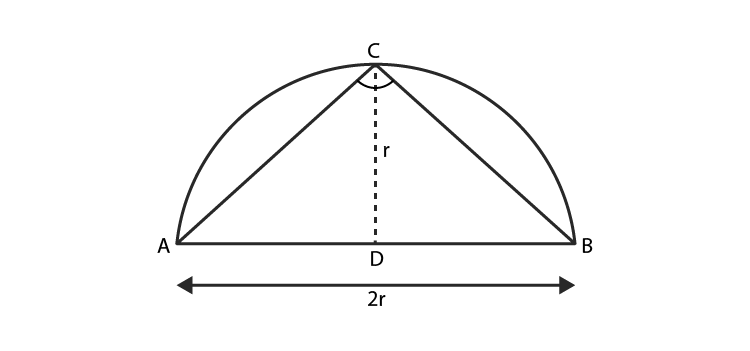∴ ∠ C = 90° (by the properties of circle)

So, ΔABC is right angled triangle with base as diameter AB of the circle and height be CD.

Height of the triangle = r

∴ Area of largest ΔABC = (1/2)× Base × Height = (1/2)× AB × CD

= (1/2)× 2r × r = r2 sq. units

∴ Option A is correct.

5. If the perimeter of a circle is equal to that of a square, then the ratio of their areas is

(A) 22 : 7 (B) 14 : 11 (C) 7 : 22 (D) 11: 14

Solution:

(B) 14 : 11

Explanation:

Let r be the radius of the circle and a be the side of the square.

According to the question,

Perimeter of a circle = Perimeter of a square

⇒ 2πr = 4a

⇒ a = πr/2

Area of the circle = r2 and Area of the square = a2

Now, Ratio of their areas = (Area of circle)/(Area of square)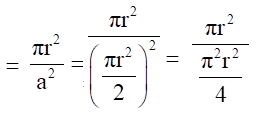= 4/π

= [4/(22/7)]

= 14/11

∴ Option B is correct.

## Exercise 11.2 Page No: 122

1. Is the area of the circle inscribed in a square of side a cm, a2 cm2? Give reasons for your answer.

Solution:

False

Explanation:

Let a be the side of square.

We are given that the circle is inscribed in the square.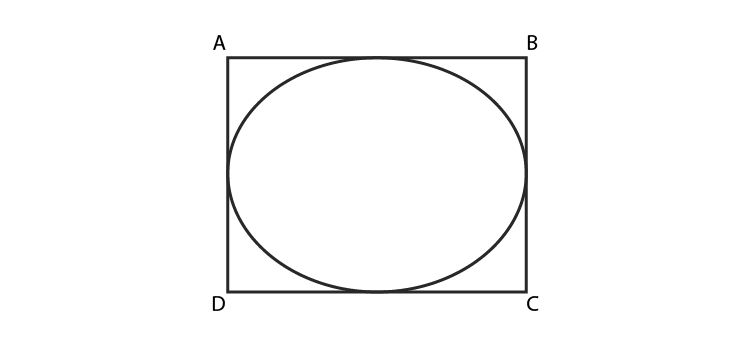Diameter of circle = Side of square = a

Radius of the circle = a/2

Area of the circle = πr2 = π(a/2)2 = (πa2)/4 cm2

Hence, area of the circle is (πa2)/4 cm2

Thus the area of the circle inscribed in a square of side a cm is not a2 cm2

2. Will it be true to say that the perimeter of a square circumscribing a circle of radius a cm is 8a cm? Give reasons for your answer.

Solution:

True

Explanation:

Let r be the radius of circle = a cm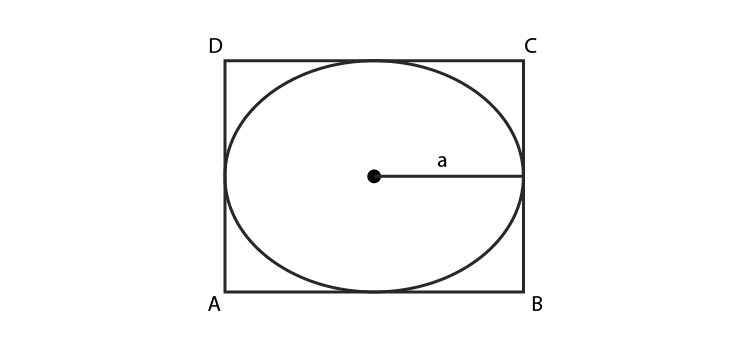∴ Diameter of the circle = d = 2 × Radius = 2a cm

As the circle is inscribed in the square, therefore,

Side of a square = Diameter of circle = 2a cm

Hence, Perimeter of a square = 4 × (side) = 4 × 2a = 8a cm

Thus, it will be true to say that the perimeter of a square circumscribing a circle of radius a cm is 8a cm.

3. In Fig 11.3, a square is inscribed in a circle of diameter d and another square is circumscribing the circle. Is the area of the outer square four times the area of the inner square? Give reasons for your answer.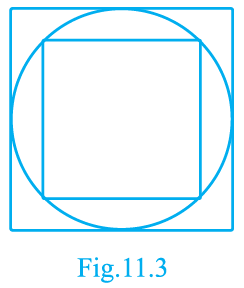Solution:

False

Explanation:

Diameter of the circle = d

Therefore,

Diagonal of inner square EFGH = Side of the outer square ABCD = Diameter of circle = d

Let side of inner square EFGH be a

Now in right angled triangle EFG,

(EG)2 = (EF)2 + (FG)2

By Pythagoras theorem)

⇒ d2 = a2 +a2

⇒ d2 = 2a2

⇒ a2 = d2/2

∴ Area of inner circle = a2 = d2/2

Also, Area of outer square = d2

∴ the area of the outer circle is only two times the area of the inner circle.

Thus, area of outer square is not equal to four times the area of the inner square.

4. Is it true to say that area of a segment of a circle is less than the area of its corresponding sector? Why?

Solution:

False

Explanation:

It is not true because in case of major segment, area is always greater than the area of its corresponding sector. It is true only in the case of minor segment.

Thus, we can conclude that it is not true to say that area of a segment of a circle is less than the area of its corresponding sector.

5. Is it true that the distance travelled by a circular wheel of diameter d cm in one revolution is 2 d cm? Why?

Solution:

False

Explanation:

Distance travelled by a circular wheel of radius r in one revolution equals the circumference of the circle.

We know that,

Circumference of the circle = 2πd; where d is the diameter of the circle.

Thus, it is not true that the distance travelled by a circular wheel of diameter d cm in one revolution is 2 d cm.

6. In covering a distance s metres, a circular wheel of radius r metres makes s/2πr revolutions. Is this statement true? Why?

Solution:

True

Explanation:

The distance travelled by a circular wheel of radius r m in one revolution is equal to the circumference of the circle = 2πr

No. of revolutions completed in 2πr m distance = 1

No. of revolutions completed in 1 m distance = (1/2πr)

No. of revolutions completed in s m distance = (1/2πr) × s = s/2πr

Thus, the statement “in covering a distance s metres, a circular wheel of radius r metres makes s/2πr revolutions” is true.

## Exercise 11.3 Page No: 125

1. Find the radius of a circle whose circumference is equal to the sum of the circumferences of two circles of radii 15 cm and 18 cm.

Solution:

Radius of first circle = r1 = 15 cm

Radius of second circle = r2 = 18 cm

∴ Circumference of first circle = 2πr1 = 30π cm

Circumference of second circle = 2πr2 = 36π cm

Let the radius of the circle = R

According to the question,

Circumference of circle = Circumference of first circle + Circumference of second circle

2πR= 2πr1+ 2πr2

⇒ 2πR = 30π + 36π

⇒ 66π ⇒ R = 33

Hence, required radius of a circle is 33 cm.

2. In Fig. 11.5, a square of diagonal 8 cm is inscribed in a circle. Find the area of the shaded region.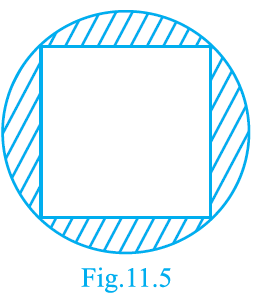Solution:

Let a be the side of square.

∴ Diameter of a circle = Diagonal of the square = 8 cm

In right angled triangle ABC,

Using Pythagoras theorem,

(AC)2 = (AB)2 + (BC)2

∴ (8)2= a2 +a2

⇒ 64= 2a2

⇒ a2= 32

Hence,

area of square = a2= 32 cm2

∴ Radius of the circle = Diameter/2 = 4 cm

∴ Area of the circle = πr2 = π(4)2 = 16 cm2

Therefore, the area of the shaded region = Area of circle – Area of square

The area of the shaded region = 16π – 32

= 16 × (22/7) – 32

= 128/7

= 18.286 cm2

3. Find the area of a sector of a circle of radius 28 cm and central angle 45°.

Solution:

Area of a sector of a circle = (1/2)r2θ,

(Here r = radius and θ = angle in radians subtended by the arc at the center of the circle)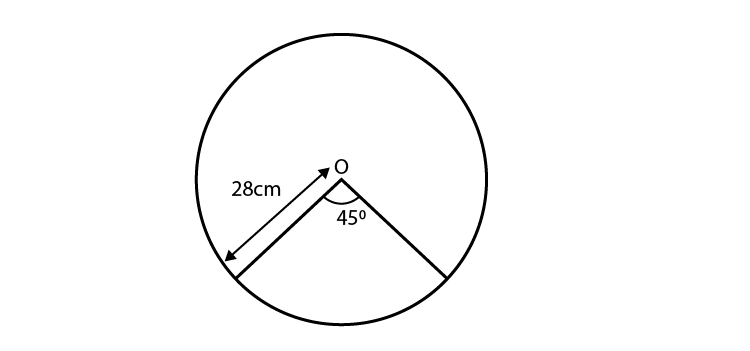Here, Radius of circle = 28 cm

Angle subtended at the center = 45°

Angle subtended at the center (in radians) = θ 45π/180 = π/4

∴ Area of a sector of a circle = ½ r2θ

= ½ × (28)2 × (π/4)

= 28 × 28 × (22/8×7)

= 308 cm2

Hence, the required area of a sector of a circle is 308 cm2.

4. The wheel of a motor cycle is of radius 35 cm. How many revolutions per minute must the wheel make so as to keep a speed of 66 km/h?

Solution:

Radius of wheel = r = 35 cm

1 revolution of the wheel = Circumference of the wheel

= 2πr

= 2 × (22/7) × 35

= 220 cm

But, given that,

Speed of the wheel = 66 km/hr

= (66×1000×100)/60 cm/min

= 110000 cm/min

∴ Number of revolutions in 1 min = 110000/220 = 500

Hence, required number of revolutions per minute is 500.

5. A cow is tied with a rope of length 14 m at the corner of a rectangular field of dimensions 20m × 16m. Find the area of the field in which the cow can graze.

Solution:

Let ABCD be a rectangular field.

Length of field = 20 m

Breadth of the field = 16 m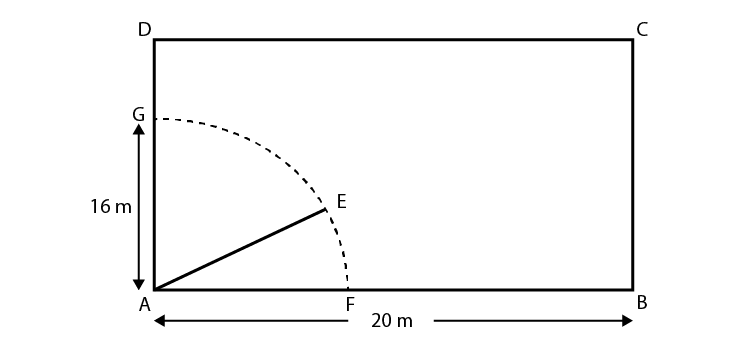According to the question,

A cow is tied at a point A.

Let length of rope be AE = 14 m = l.

Angle subtended at the center of the sector = 90°

Angle subtended at the center (in radians) θ = 90π/180 = π/2

∴ Area of a sector of a circle = ½ r2θ

= ½ × (14)2 × (π/2)

= 154 m2

Hence, the required area of a sector of a circle is 154 m2.

6. Find the area of the flower bed (with semi-circular ends) shown in Fig. 11.6.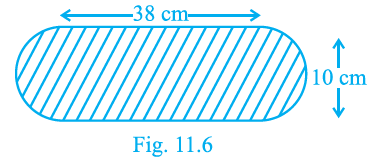Solution:

According to the given figure,

Length and breadth of the rectangular portion AFDC of the flower bed are 38 cm and 10 cm respectively.

We know that,

Area of the flower bed = Area of the rectangular portion + Area of the two semi-circles.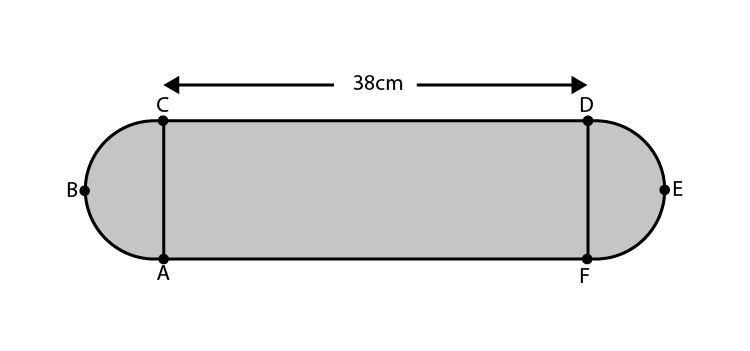∴ Area of rectangle AFDC = Length × Breadth

= 38 × 10 = 380 cm2

Both ends of flower bed are semi-circle in shape.

∴ Diameter of the semi-circle = Breadth of the rectangle AFDC = 10 cm

∴ Radius of the semi circle = 10/2 = 5 cm

Area of the semi-circle = πr2/2 = 25π/2 cm2

Since there are two semi-circles in the flower bed,

∴ Area of two semi-circles = 2 × (πr2/2) = 25π cm2

Total area of flower bed = (380 + 25π) cm2

7. In Fig. 11.7, AB is a diameter of the circle, AC = 6 cm and BC = 8 cm. Find the area of the shaded region (Use π= 3.14).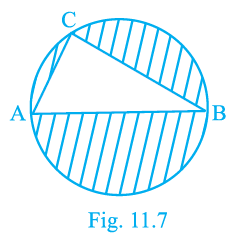Solution:

According to the question,

AC = 6cm and BC = 8 cm

A triangle in a semi-circle with hypotenuse as diameter is right angled triangle.

Using Pythagoras theorem in right angled triangle ACB,

(AB)2 = (AC)2 + (CB)2

(AB)2 = (6)2 + (8)2

⇒(AB)2 = 36 + 64

⇒(AB)2 = 100 ⇒(AB)= 10

∴ Diameter of the circle = 10 cm

Thus, Radius of the circle = 5 cm

Area of circle = πr2

= π(5)2

= 25π cm2

= 25 × 3.14 cm2

= 78.5 cm2

We know that,

Area of the right angled triangle = ( ½ ) × Base × Height

= (½) × AC × CB

= (½) × 6 × 8

= 24 cm2

Now, Area of the shaded region = Area of the circle – Area of the triangle

= (78.5-24)cm2

= 54.5cm2

## Exercise 11.4 Page No: 132

1. The area of a circular playground is 22176 m2. Find the cost of fencing this ground at the rate of Rs 50 per metre.

Solution:

According to the question,

Area of the circular playground = 22176 m2 (Given)

Let r be the radius of the circle.

∴ πr2 = 22176

⇒ (22/7)r2 = 22176

⇒ r2 = 22176 × (22/7)

⇒ r2 = 7056

⇒ r = 84

∴ Radius of the circular playground = 84 m

Now, circumference of the circle = 2πr

= 2×(22/7)×84

= 528 m

Cost of fencing 1 meter of ground = Rs 50

∴ Cost of fencing the total ground = Rs 528 × 50 = Rs 26,400

2. The diameters of front and rear wheels of a tractor are 80 cm and 2 m respectively. Find the number of revolutions that rear wheel will make in covering a distance in which the front wheel makes 1400 revolutions.

Solution:

According to the question,

Diameter of front wheels = d1 = 80 cm

Diameter of rear wheels = d2 = 2 m = 200 cm

Let r1 be the radius of the front wheels = 80/2 = 40 cm

Let r2 be the radius of the rear wheels = 200/2 = 100 cm

Now, Circumference of the front wheels = 2πr

= 2 ×(22/7) × 40

= 1760/7 cm

Circumference of the rear wheels = 2πr = 2 × (22/7) × 100 = 4400/7 cm

No. of revolutions made by the front wheel = 1400

∴ Distance covered by the front wheel = 1400 × (1760/7) = 352000 cm

Number of revolutions made by rear wheel in covering a distance in which the front wheel makes 1400 revolutions,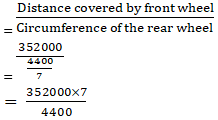= 560 revolutions.

3. Sides of a triangular field are 15 m, 16 m and 17 m. With the three corners of the field a cow, a buffalo and a horse are tied separately with ropes of length 7 m each to graze in the field. Find the area of the field which cannot be grazed by the three animals.

Solution:

According to the question,

Sides of the triangle are 15 m, 16 m, and 17 m.

Now, perimeter of the triangle = (15+16+17) m = 48 m

∴ Semi-perimeter of the triangle = s = 48/2 = 24 m

By Heron’s formula,

Area of the triangle = √(s(s – a)(s – b)(s – c)) ,here a, b and c are the sides of triangle

= √(24(24 – 15)(24 – 16)(24 – 17))

= 109.982 m2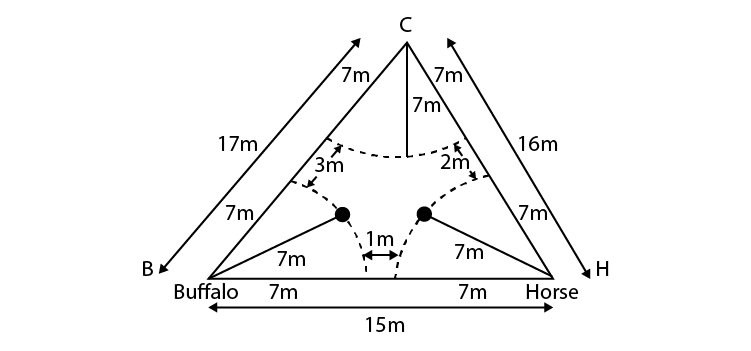Let B, C and H be the corners of the triangle on which buffalo, cow and horse are tied respectively with ropes of 7 m each.

So, the area grazed by each animal will be in the form of a sector.

∴ Radius of each sector = r = 7 m

Let x, y, z be the angles at corners B, C, H respectively.

∴ Area of sector with central angle x,

= ½ (x/180) × πr2 = (x/360) × π(7)2

Area of sector with central angle y,

= ½ (y/180) × πr2 = (y/360) × π(7)2

Area of sector with central angle z,

= ½ (z/180) × πr2 = (z/360) × π(7)2

Area of field not grazed by the animals = Area of triangle – (area of the three sectors)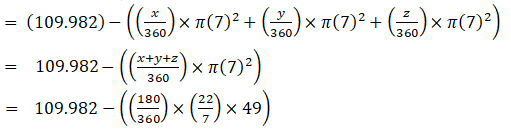= 109.892 – 77

= 32.982 cm2

4. Find the area of the segment of a circle of radius 12 cm whose corresponding sector has a central angle of 60° (Use π = 3.14).

Solution:

According to the question,

Radius of the circle = r = 12 cm

∴ OA = OB = 12 cm

∠ AOB = 60° (given)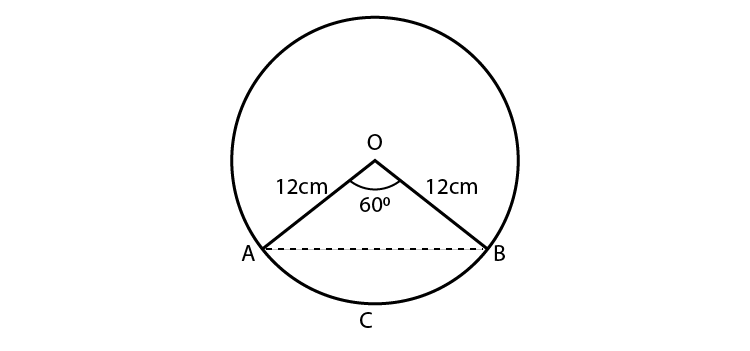Since triangle OAB is an isosceles triangle, ∴ ∠ OAB = ∠ OBA = θ (say)

Also, Sum of interior angles of a triangle is 180°,

∴ θ + θ + 60° = 180°

⇒2θ = 120° ⇒ θ = 60°

Thus, the triangle AOB is an equilateral triangle.

∴ AB = OA = OB = 12 cm

Area of the triangle AOB = (√3 /4)× a2,where a is the side of the triangle.

= (√3 /4) × (12)2

= (√3 /4) × 144

= 36√3 cm2

= 62.354 cm2

Now, Central angle of the sector AOBCA = ∅ = 60° =  (60π / 180) = (π/3) radians

Thus, area of the sector AOBCA = ½ r2

= ½ × 122 × π/3

= 122 × (22 / (7×6))

= 75.36 cm2

Now, Area of the segment ABCA = Area of the sector AOBCA – Area of the triangle AOB

= (75.36 – 62.354) cm2 = 13.006 cm2

5. A circular pond is 17.5 m is of diameter. It is surrounded by a 2 m wide path. Find the cost of constructing the path at the rate of Rs 25 per m2

Solution:

Diameter of the circular pond = 17.5 m

Let r be the radius of the park = (17.5/2) m = 8.75 m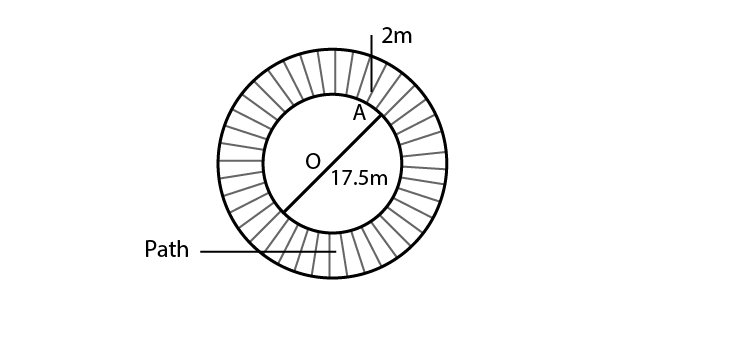According to the question,

The circular pond is surrounded by a path of width 2 m.

So, Radius of the outer circle = R = (8.75+2) m = 10.75 m

Area of the road = Area of the outer circular path – Area of the circular pond

= πr2 – πR2

= 3.14 × (10.75)2 – 3.14 × (8.75)2

= 3.14 × ((10.75)2 – (8.75)2)

= 3.14 × ((10.75 + 8.75) × (10.75 – 8.75))

= 3.14 × 19.5 × 2 = 122.46 m2

Hence, the area of the path is 122.46 m2.

Now, Cost of constructing the path per m2 = Rs. 25

∴ cost of constructing 122.46m2 of the path = Rs. 25 × 122.46 = Rs. 3061.50

6. In Fig. 11.17, ABCD is a trapezium with AB || DC, AB = 18 cm, DC = 32 cm and distance between AB and DC = 14 cm. If arcs of equal radii 7 cm with centres A, B, C and D have been drawn, then find the area of the shaded region of the figure.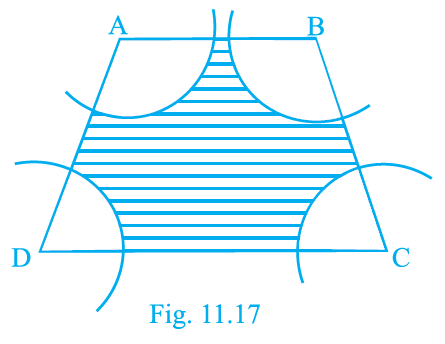Solution:

AB = 18 cm, DC = 32 cm

Distance between AB and DC = Height = 14 cm

Now, Area of the trapezium = (1/2) × (Sum of parallel sides) × Height

= (½) × (18+32) × 14 = 350cm2

As AB ∥ DC, ∴ ∠ A +∠ D = 180°

And ∠ B +∠ C = 180°

Also, radius of each arc = 7 cm

Therefore,

Area of the sector with central angle A = (½) × (∠A/180) × π × r2

Area of the sector with central angle D = (½) × (∠D/180) × π × r2

Area of the sector with central angle B = (½) × (∠B/180) × π × r2

Area of the sector with central angle C = (½) × (∠C/180) × π × r2

Total area of the sectors,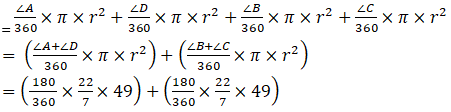= 77 + 77

= 154

∴ Area of shaded region = Area of trapezium – (Total area of sectors)

= 350 – 154 = 196 cm2

Hence, the required area of shaded region is 196 cm2.

7. Three circles each of radius 3.5 cm are drawn in such a way that each of them touches the other two. Find the area enclosed between these circles.

Solution:

The three circles are drawn such that each of them touches the other two.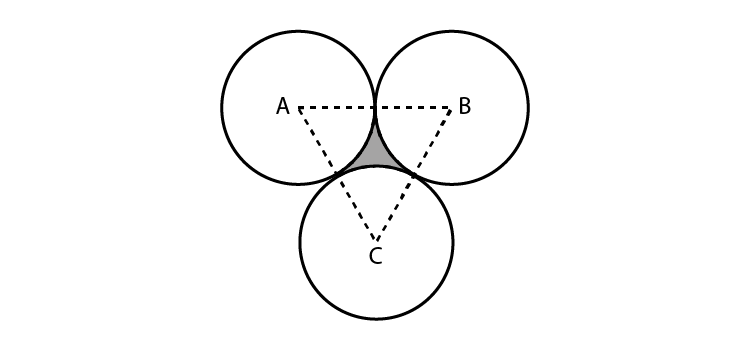By joining the centers of the three circles,

We get,

AB = BC = CA = 2(radius) = 7 cm

Therefore, triangle ABC is an equilateral triangle with each side 7 cm.

∴ Area of the triangle = (√3 /4) × a2, where a is the side of the triangle.

= (√3 /4) × 72

= (49/4) √3 cm2

= 21.2176 cm2

Now, Central angle of each sector = ∅ = 60° (60π/180)

Thus, area of each sector = (1/2) r2θ

= (1/2) × (3.5)2 × (π/3)

= 12.25 × (22/ (7×6))

= 6.4167 cm2

Total area of three sectors = 3 × 6.4167 = 19.25 cm2

∴ Area enclosed between three circles = Area of triangle ABC – Area of the three sectors

= 21.2176 – 19.25

= 1.9676 cm2

Hence, the required area enclosed between these circles is 1.967 cm2 (approx.).

8. Find the area of the sector of a circle of radius 5 cm, if the corresponding arc length is 3.5 cm.

Solution: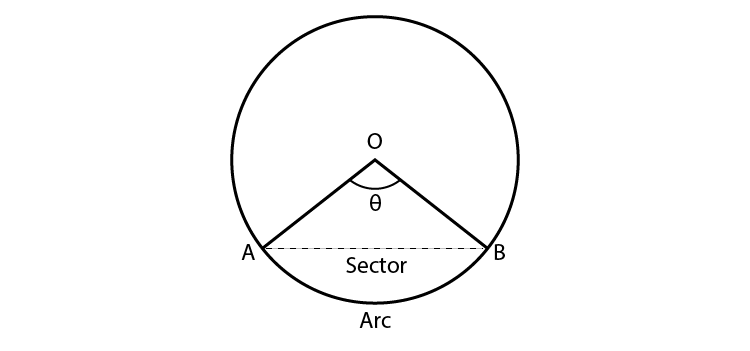Radius of the circle = r = 5 cm

Arc length of the sector = l = 3.5 cm

Let the central angle (in radians) be θ.

∴ Central angle (θ) = Arc length / Radius = l / r = 3.5/5 = 0.7 radians

Now, Area of the sector = (½) × r2θ = (½) × 25 × 0.7 = 8.75 cm2

Hence, required area of the sector of a circle is 8.75 cm2.

9. Four circular cardboard pieces of radii 7 cm are placed on a paper in such a way that each piece touches other two pieces. Find the area of the portion enclosed between these pieces.

Solution:

According to the question,

The four circles are placed such that each piece touches the other two pieces.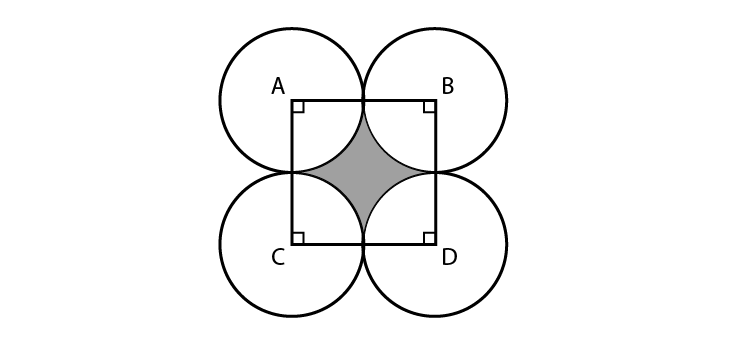By joining the centers of the circles by a line segment, we get a square ABDC with sides,

AB = BD = DC = CA = 2(Radius) = 2(7) cm = 14 cm

Now, Area of the square = (Side)2 = (14)2 = 196 cm2

ABDC is a square,

Therefore, each angle has a measure of 90°.

i.e., ∠ A = ∠ B = ∠ D = ∠ C = 90° = π/2 radians = θ (say)

Given that,

Radius of each sector = 7 cm

Area of the sector with central angle A = (½)r2θ

= ½ r2 θ

= ½ × 49 × π/2

= ½ × 49 × (22/(2×7))

= (77/2) cm2

Since the central angles and the radius of each sector are same, area of each sector is 77/2 cm2

∴ Area of the shaded portion = Area of square – Area of the four sectors

= 196 – (4 × (77/2))

= 196 – 154

= 42 cm2

Therefore, required area of the portion enclosed between these pieces is 42 cm2.

10. On a square cardboard sheet of area 784 cm2, four congruent circular plates of maximum size are placed such that each circular plate touches the other two plates and each side of the square sheet is tangent to two circular plates. Find the area of the square sheet not covered by the circular plates.

Solution:

According to the question,

Area of the square = 784 cm2

∴ Side of the square = √Area = √784 = 28 cm

The four circular plates are congruent,

Then diameter of each circular plate = 28/2 = 14 cm

∴ Radius of each circular plate = 7 cm

We know that,

Area of the sheet not covered by plates = Area of the square – Area of the four circular plates

As all four circular plates are congruent,

We have, area of all four plates equal.

∴ Area of one circular plate = πr2 = (22/7) × 72 = 154 cm2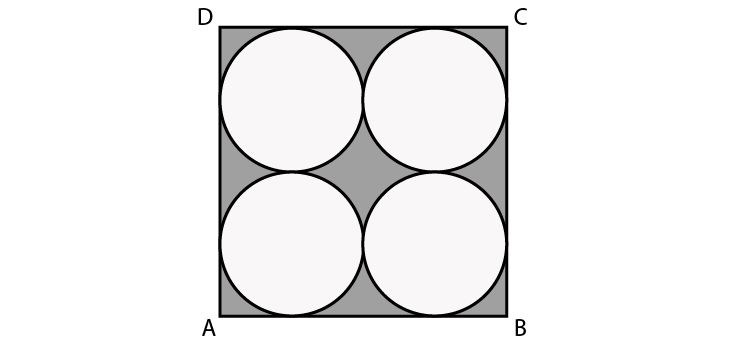Then,

Area of four plates = 4×154 = 616 cm2

Area of the sheet not covered by plates = 784 – 616 = 168 cm2

The Chapter “Areas Related to Circles”, given in the Class 10 Maths textbook has been specially put up together to help students learn the main concepts of the perimeter (circumference) as well as the area of a circle. At the end of the chapter, students will have to apply their theoretical understanding of the whatever they have learned to basically solve problems related to areas of circles, its segment, sector as well as combinations of plane figures.

While the sums in the chapter can be quite tricky, we are providing free NCERT Exemplar for Chapter 11 here. Online learning materials such as notes, exemplar books, NCERT Maths Solutions for Class 10 and question papers are also provided here for 10th standard students to make them prepared for the board exams. Although the sample papers and previous year question papers are of great help to get through the idea of types of questions asked in the exam from this chapter and marks carried by it.

Download BYJU’S app to get personalized videos, explaining different types of Maths topics such as area and perimeter of the circle, triangles, etc. and experience a new approach of learning to understand the concepts in an easy way.

## Frequently Asked Questions on NCERT Exemplar Solutions for Class 10 Maths Chapter 11

### What is a segment of a circle in NCERT Exemplar Solutions for Class 10 Maths Chapter 11?

A segment of a circle can be defined as a region bounded by a chord and a corresponding arc lying between the chord’s endpoints. In other words, a circular segment is a region of a circle which is created by breaking apart from the rest of the circle through a secant or a chord. We can also define segments as the parts that are divided by the circle’s arc and connected through a chord by the endpoints of the arc. It is to be noted that the segments do not contain the center point.

### What are the important topics covered in Chapter 11 of NCERT Exemplar Solutions for Class 10 Maths?

The important topics covered in NCERT Exemplar Solutions for Class 10 Maths Chapter 11 are:
1. Finding the Perimeter and Area of a Circle
2. Finding the area of the sector and segment of a circle
3. Finding the areas of Combinations of Plane Figures

### Is NCERT Exemplar Solutions for Class 10 Maths Chapter 11 the best study material for the students during revision?

Yes, the NCERT Exemplar Solutions for Class 10 Maths Chapter 11 is the best study material which helps students to revise the complex concepts effortlessly. Each solution provides a logical explanation to make learning easier for the students. The in-house team of experts at BYJU’S have framed the step wise solutions to encourage the analytical thinking approach among students. These solutions can also be compared in order to get an idea about the other methods which can be used to solve the textbook problems.